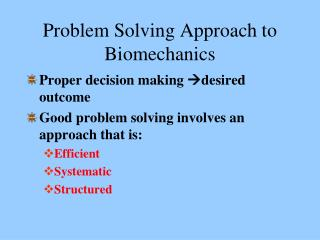DownloadDownload PresentationProblem Solving Approach to Biomechanics

# Problem Solving Approach to Biomechanics

Download Presentation## Problem Solving Approach to Biomechanics

- - - - - - - - - - - - - - - - - - - - - - - - - - - E N D - - - - - - - - - - - - - - - - - - - - - - - - - - -
##### Presentation Transcript

1. Problem Solving Approach to Biomechanics Proper decision makingdesired outcome Good problem solving involves an approach that is: Efficient Systematic Structured

2. Types of Analysis • Qualitative – Involves a non-numerical description. “The boulder is heavy.” • Quantitative – Involves the use of numbers. “The boulder weighs 750 pounds.”

3. Solving Qualitative Problems • Is the movement being performed with proper or adequate force? • Is the movement being performed through an appropriate range of motion? • Is the sequence (or pattern) of body movements appropriate (or optimal) for the execution of the skill? • Qualitative data may be collected based on: • Observation • Knowledge of skill technique

4. Formal Problem Solving Method • Given a set of information or data EXAMPLE: An ACL requiring reconstruction • Establish a goal or desired result EXAMPLE: Regain strength, stability, and range of motion • Implement a procedure or process to achieve the goal EXAMPLE: Surgery followed by therapeutic rehabilitation

5. Format for Quantitative Problem Solving GIVEN: List the known data and conditions. (Write down what you know, including implied information.) FIND: Formally state the goal of the solution. DIAGRAM: Pictorially represent the problem (when this is practical). FORMULAS: List the formulas to be used and any inferred or derived information. SOLUTION: Actual steps in solving the problem. Solve for unknown variables. ANSWER: Make sure that this is expressed in the appropriate units.

6. GIVEN: A car driving at an average velocity of 30 MPH (or 30 miles/hr) FIND: The distance the car will travel in 83 minutes DIAGRAM: FORMULAS: velocity = distance/time Therefore, distance = velocity*time 1 hour = 60 minutes SOLUTION: Time = (83 minutes)(1 hr/60 minutes) = 1.38 hrs distance = (30 miles/hr)(1.38 hrs) ANSWER: distance = 41.4 miles V = 30 miles/hr t = 0 min t = 83 min distance